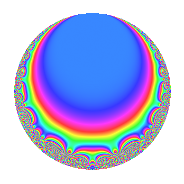# Properties

 Label 23.3.b.aLevel 23 Weight 3 Character orbit 23.b Self dual Yes Analytic conductor 0.627 Analytic rank 0 Dimension 3 CM disc. -23 Inner twists 2

# Related objects

## Newspace parameters

 Level: $$N$$ = $$23$$ Weight: $$k$$ = $$3$$ Character orbit: $$[\chi]$$ = 23.b (of order $$2$$ and degree $$1$$)

## Newform invariants

 Self dual: Yes Analytic conductor: $$0.626704608029$$ Analytic rank: $$0$$ Dimension: $$3$$ Coefficient field: 3.3.621.1 Coefficient ring: $$\Z[a_1, a_2, a_3]$$ Coefficient ring index: $$1$$ Sato-Tate group: $\mathrm{U}(1)[D_{2}]$

## $q$-expansion

Coefficients of the $$q$$-expansion are expressed in terms of a basis $$1,\beta_1,\beta_2$$ for the coefficient ring described below. We also show the integral $$q$$-expansion of the trace form.

 $$f(q)$$ $$=$$ $$q + ( \beta_{1} + \beta_{2} ) q^{2} + ( -2 \beta_{1} - \beta_{2} ) q^{3} + ( 4 + \beta_{1} - 2 \beta_{2} ) q^{4} + ( -11 - 3 \beta_{1} + 2 \beta_{2} ) q^{6} + ( -7 + 4 \beta_{1} + 4 \beta_{2} ) q^{8} + ( 9 + 6 \beta_{1} - \beta_{2} ) q^{9} +O(q^{10})$$ $$q + ( \beta_{1} + \beta_{2} ) q^{2} + ( -2 \beta_{1} - \beta_{2} ) q^{3} + ( 4 + \beta_{1} - 2 \beta_{2} ) q^{4} + ( -11 - 3 \beta_{1} + 2 \beta_{2} ) q^{6} + ( -7 + 4 \beta_{1} + 4 \beta_{2} ) q^{8} + ( 9 + 6 \beta_{1} - \beta_{2} ) q^{9} + ( 1 - 11 \beta_{1} - 11 \beta_{2} ) q^{12} + ( -2 \beta_{1} + 7 \beta_{2} ) q^{13} + ( 16 - 7 \beta_{1} - 7 \beta_{2} ) q^{16} + ( 13 + 22 \beta_{1} + 11 \beta_{2} ) q^{18} -23 q^{23} + ( -44 + 2 \beta_{1} + 15 \beta_{2} ) q^{24} + 25 q^{25} + ( 29 - 11 \beta_{1} - 14 \beta_{2} ) q^{26} + ( -38 - 18 \beta_{1} - 9 \beta_{2} ) q^{27} + ( -2 \beta_{1} - 17 \beta_{2} ) q^{29} + ( 22 \beta_{1} + 7 \beta_{2} ) q^{31} + ( -28 - 7 \beta_{1} + 14 \beta_{2} ) q^{32} + ( 85 + 22 \beta_{1} - 5 \beta_{2} ) q^{36} + ( -14 + 6 \beta_{1} + 23 \beta_{2} ) q^{39} + ( -26 \beta_{1} + 7 \beta_{2} ) q^{41} + ( -23 \beta_{1} - 23 \beta_{2} ) q^{46} + ( 22 \beta_{1} - 17 \beta_{2} ) q^{47} + ( 77 - 11 \beta_{1} - 30 \beta_{2} ) q^{48} + 49 q^{49} + ( 25 \beta_{1} + 25 \beta_{2} ) q^{50} + ( -103 + 29 \beta_{1} + 29 \beta_{2} ) q^{52} + ( -99 - 65 \beta_{1} - 20 \beta_{2} ) q^{54} + ( -91 + 13 \beta_{1} + 34 \beta_{2} ) q^{58} + 26 q^{59} + ( 101 + 37 \beta_{1} - 14 \beta_{2} ) q^{62} + ( -15 - 28 \beta_{1} - 28 \beta_{2} ) q^{64} + ( 46 \beta_{1} + 23 \beta_{2} ) q^{69} + ( -26 \beta_{1} + 31 \beta_{2} ) q^{71} + ( -11 + 46 \beta_{1} + 51 \beta_{2} ) q^{72} + ( -26 \beta_{1} - 41 \beta_{2} ) q^{73} + ( -50 \beta_{1} - 25 \beta_{2} ) q^{75} + ( 133 - 25 \beta_{1} - 60 \beta_{2} ) q^{78} + ( 81 + 76 \beta_{1} + 38 \beta_{2} ) q^{81} + ( -43 - 59 \beta_{1} - 14 \beta_{2} ) q^{82} + ( 82 + 6 \beta_{1} - 49 \beta_{2} ) q^{87} + ( -92 - 23 \beta_{1} + 46 \beta_{2} ) q^{92} + ( -182 - 66 \beta_{1} - \beta_{2} ) q^{93} + ( -19 + 61 \beta_{1} + 34 \beta_{2} ) q^{94} + ( -7 + 77 \beta_{1} + 77 \beta_{2} ) q^{96} + ( 49 \beta_{1} + 49 \beta_{2} ) q^{98} +O(q^{100})$$ $$\operatorname{Tr}(f)(q)$$ $$=$$ $$3q + 12q^{4} - 33q^{6} - 21q^{8} + 27q^{9} + O(q^{10})$$ $$3q + 12q^{4} - 33q^{6} - 21q^{8} + 27q^{9} + 3q^{12} + 48q^{16} + 39q^{18} - 69q^{23} - 132q^{24} + 75q^{25} + 87q^{26} - 114q^{27} - 84q^{32} + 255q^{36} - 42q^{39} + 231q^{48} + 147q^{49} - 309q^{52} - 297q^{54} - 273q^{58} + 78q^{59} + 303q^{62} - 45q^{64} - 33q^{72} + 399q^{78} + 243q^{81} - 129q^{82} + 246q^{87} - 276q^{92} - 546q^{93} - 57q^{94} - 21q^{96} + O(q^{100})$$

Basis of coefficient ring in terms of a root $$\nu$$ of $$x^{3} - 6 x - 3$$:

 $$\beta_{0}$$ $$=$$ $$1$$ $$\beta_{1}$$ $$=$$ $$\nu$$ $$\beta_{2}$$ $$=$$ $$\nu^{2} - \nu - 4$$
 $$1$$ $$=$$ $$\beta_0$$ $$\nu$$ $$=$$ $$\beta_{1}$$ $$\nu^{2}$$ $$=$$ $$\beta_{2} + \beta_{1} + 4$$

## Character Values

We give the values of $$\chi$$ on generators for $$\left(\mathbb{Z}/23\mathbb{Z}\right)^\times$$.

 $$n$$ $$5$$ $$\chi(n)$$ $$-1$$

## Embeddings

For each embedding $$\iota_m$$ of the coefficient field, the values $$\iota_m(a_n)$$ are shown below.

For more information on an embedded modular form you can click on its label.

Label $$\iota_m(\nu)$$ $$a_{2}$$ $$a_{3}$$ $$a_{4}$$ $$a_{5}$$ $$a_{6}$$ $$a_{7}$$ $$a_{8}$$ $$a_{9}$$ $$a_{10}$$
22.1
 −0.523976 −2.14510 2.66908
−3.72545 4.24943 9.87897 0 −15.8310 0 −21.9018 9.05761 0
22.2 0.601466 1.54364 −3.63824 0 0.928445 0 −4.59414 −6.61718 0
22.3 3.12398 −5.79306 5.75927 0 −18.0974 0 5.49593 24.5596 0
 $$n$$: e.g. 2-40 or 990-1000 Significant digits: Format: Complex embeddings Normalized embeddings Satake parameters Satake angles

## Inner twists

Char. orbit Parity Mult. Self Twist Proved
1.a Even 1 trivial yes
23.b Odd 1 CM by $$\Q(\sqrt{-23})$$ yes

## Hecke kernels

There are no other newforms in $$S_{3}^{\mathrm{new}}(23, [\chi])$$.